# Resolution

When digitizing the samples, the voltage at each sample time is converted to a number. This is done by comparing the voltage with a number of levels. The resulting number is the number of the highest level that's still lower than the voltage. The number of levels is determined by the resolution. The higher the resolution, the more levels are available and the more accurate the input signal can be reconstructed. In the image below, the same signal is digitized, using three different amounts of levels: 16, 32 and 64.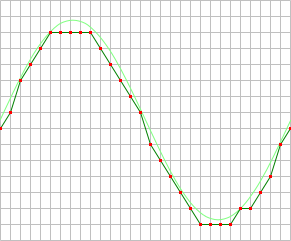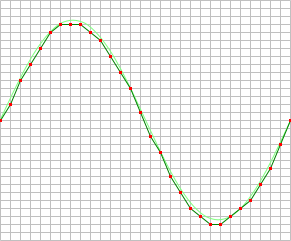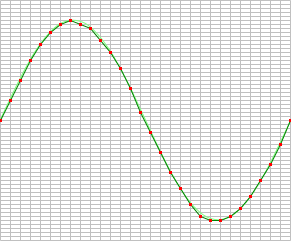The number of available levels is determined by the resolution: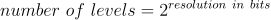The used resolutions in the previous image are respectively: 4 bits, 5 bits and 6 bits.

The smallest detectable voltage difference depends on the resolution and the input range. This voltage can be calculated as: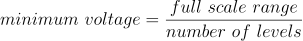In the 200 mV range, the full scale ranges from -200 mV to +200 mV, the full range is 400 mV. When a 12 bit resolution is used, there are 212 = 4096 levels. This results in a smallest detectable voltage step of 0.400 V / 4096 = 97.7 µV. In 16 bit resolution this step is 0.400 V / 65536 = 6.1 µV

Changing the resolution of an instrument in the Multi Channel oscilloscope software can be done in various different ways:

• Right-clicking the instrument in the Object Tree, selecting Resolution and then the appropriate resolution value in the popup menu
• Clicking the decrease/increase resolution buttons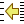and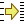on the instrument toolbar
• Clicking the resolution indicator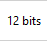on the instrument toolbar and selecting the required resolution from the popup menu